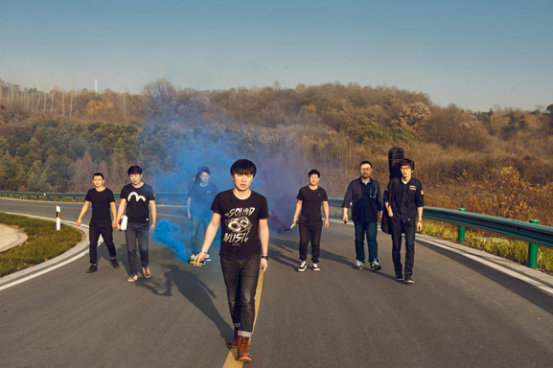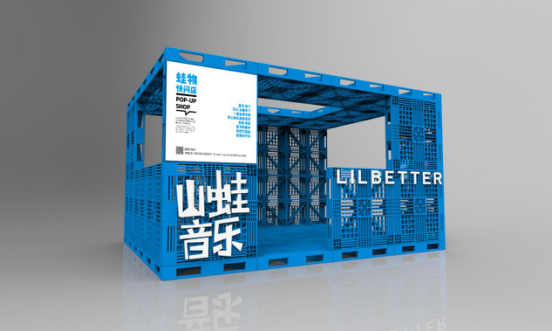|

# 跟着奥克斯空调山蛙音乐节的节奏造作起来

作为一直领跑空调产业发展变革与消费潮流的领头羊，奥克斯空调凭借对市场以及消费群体的精准把握能力，创新品牌营销模式，大胆求变，采用娱乐营销、用户全民体验营销等新形式，推出属于自己的健康时尚体育跑、“奥粉节”，冠名山蛙音乐节，成为家电行业的“时尚教主”。

在今年6月份，奥克斯空调秉承着原创的理念与年轻且活力的山蛙音乐强强联手，打造最具态度的“奥克斯空调山蛙音乐节”,计划2017年内推出十场不同主题的音乐派对。其中，以“蛙奥，无态度不青春”为主题首场音乐派对6月5日在杭州宝龙城市广场狂热开场，获得无数年轻人的“点赞”。 据悉，在7月2日奥克斯空调联手天猫、山蛙音乐打造的主题为“属于夏天的100只西呱”的第二场音乐派对即将清凉来袭，邀您相约杭州城西银泰，那就让我们提前感受下它的“态度”。为了准备第二场音乐派对，奥克斯空调与山蛙音乐在创意点上都下足了功夫。正值炎炎夏日，西瓜与空调是人们解暑的最佳良品，奥克斯空调抓住这一点，将西瓜元素融入到音乐派对，联手山蛙音乐打造一场充满趣味的西瓜音乐派对。奥克斯空调的创意总会颠覆你的眼球， 西瓜气球、西瓜扇子、西瓜发箍等可爱的小物件充斥在整个音乐节现场，将小伙伴们置身于充满夏日气息的清凉世界中。然而，从场景营销来讲，奥克斯空调采用各类西瓜元素而打造出的清凉夏日音乐节，不仅可以拉近品牌与消费者的距离，更在互动过程中加深用户对品牌的认可度与满意度。奥克斯空调与山蛙音乐再度联手打造的第二场充满无限创意的“西瓜音乐派对”，从主题的确定，两者就有着惺惺相惜的契合点。

主题上，奥克斯空调从“极速侠”、“雅典娜”以及“黄金侠”三款热销机型的品牌产品特性上找到灵感，它们有着30秒速冷的功效，跟炎炎夏日形成鲜明的“温差”，但却与西瓜有着异曲同工之妙，均是夏天最佳的解暑“神器”。这个夏天最好的解暑姿势，就是上天猫奥克斯旗舰店，把解暑神器奥克斯空调搬回家。而“属于夏天的100只西呱”的主题中“西呱”的“呱”也是结合了蛙的叫声，将山蛙的形象融入到主题，凸显山蛙的独特个性。

山蛙音乐节称得上是江浙沪高校原创音乐节的NO.1，每年都会有大量原创音乐产生，“原创音乐扶植计划”更是吸引了众多爱音乐的朋友。据悉，第二场“奥克斯空调山蛙音乐节”山蛙邀请到了五支乐队，它们分别是五毫克乐队、丛竹乐队、猫鱼影子、sphinx乐队以及柠檬华尔兹。其中，一支来自江苏南京的原创乐队-五毫克乐队，备受瞩目。他们组建于2015年11月份，乐队成员分别来自7个不同的城市，乐队风格以民谣、流行摇滚为主。如此强大的演出阵容，令人满心期待，更是想同他们一起为音乐狂热造作！你能想象当“创意市集”遇上“音乐节”，会是怎样的场景么？据了解，活动现场还有城西银泰与山蛙音乐、KALA创办的音乐市集围绕在音乐节舞台的周围，以有趣的摊位单元构成的“小方阵”，展示的是每一位创意摊主的原创手艺。两者相互碰撞，强烈的化学反应使得“奥克斯空调山蛙音乐节”迸发出别样的火花。不仅如此，现场还将有“蛙物快闪店”空降城西银泰城，lilbetter领军的年轻服装品牌将在快闪店中一展风采。夜宵党的最爱“小龙虾”也将惊现现场，不仅可以从“小龙虾贩卖机”购买美味的小龙虾，还设置了钓小龙虾等趣味互动小游戏。无限创意中透露出的趣味性，第二场“奥克斯空调山蛙音乐节”还未开始就已经赚足了眼球。无论是主题、内容还是创意，奥克斯空调把目光聚焦在年轻群体上，以“接地气”的活动形式、全方位的渗透互动传播将“时尚化、年轻态”的品牌理念传递给年轻消费者，让他们在娱乐中感知品牌的活力。然而，正是因为娱乐营销手段的创新与品牌策略的升级相互碰撞，奥克斯空调释放出一股新的爆发力和创造力，完成了年轻、时尚、高端的新品牌蜕变。

`声明：本文由入驻焦点开放平台的作者撰写，除焦点官方账号外，观点仅代表作者本人，不代表焦点立场错误信息举报电话： 400-099-0099，邮箱：jubao@vip.sohu.com，或点此进行意见反馈，或点此进行举报投诉。`A B C D E F G H J K L M N P Q R S T W X Y Z
A - B - C - D - E
• A
• 鞍山
• 安庆
• 安阳
• 安顺
• 安康
• 澳门
• B
• 北京
• 保定
• 包头
• 巴彦淖尔
• 本溪
• 蚌埠
• 亳州
• 滨州
• 北海
• 百色
• 巴中
• 毕节
• 保山
• 宝鸡
• 白银
• 巴州
• C
• 承德
• 沧州
• 长治
• 赤峰
• 朝阳
• 长春
• 常州
• 滁州
• 池州
• 长沙
• 常德
• 郴州
• 潮州
• 崇左
• 重庆
• 成都
• 楚雄
• 昌都
• 慈溪
• 常熟
• D
• 大同
• 大连
• 丹东
• 大庆
• 东营
• 德州
• 东莞
• 德阳
• 达州
• 大理
• 德宏
• 定西
• 儋州
• 东平
• E
• 鄂尔多斯
• 鄂州
• 恩施
F - G - H - I - J
• F
• 抚顺
• 阜新
• 阜阳
• 福州
• 抚州
• 佛山
• 防城港
• G
• 赣州
• 广州
• 桂林
• 贵港
• 广元
• 广安
• 贵阳
• 固原
• H
• 邯郸
• 衡水
• 呼和浩特
• 呼伦贝尔
• 葫芦岛
• 哈尔滨
• 黑河
• 淮安
• 杭州
• 湖州
• 合肥
• 淮南
• 淮北
• 黄山
• 菏泽
• 鹤壁
• 黄石
• 黄冈
• 衡阳
• 怀化
• 惠州
• 河源
• 贺州
• 河池
• 海口
• 红河
• 汉中
• 海东
• 怀来
• I
• J
• 晋中
• 锦州
• 吉林
• 鸡西
• 佳木斯
• 嘉兴
• 金华
• 景德镇
• 九江
• 吉安
• 济南
• 济宁
• 焦作
• 荆门
• 荆州
• 江门
• 揭阳
• 金昌
• 酒泉
• 嘉峪关
K - L - M - N - P
• K
• 开封
• 昆明
• 昆山
• L
• 廊坊
• 临汾
• 辽阳
• 连云港
• 丽水
• 六安
• 龙岩
• 莱芜
• 临沂
• 聊城
• 洛阳
• 漯河
• 娄底
• 柳州
• 来宾
• 泸州
• 乐山
• 六盘水
• 丽江
• 临沧
• 拉萨
• 林芝
• 兰州
• 陇南
• M
• 牡丹江
• 马鞍山
• 茂名
• 梅州
• 绵阳
• 眉山
• N
• 南京
• 南通
• 宁波
• 南平
• 宁德
• 南昌
• 南阳
• 南宁
• 内江
• 南充
• P
• 盘锦
• 莆田
• 平顶山
• 濮阳
• 攀枝花
• 普洱
• 平凉
Q - R - S - T - W
• Q
• 秦皇岛
• 齐齐哈尔
• 衢州
• 泉州
• 青岛
• 清远
• 钦州
• 黔南
• 曲靖
• 庆阳
• R
• 日照
• 日喀则
• S
• 石家庄
• 沈阳
• 双鸭山
• 绥化
• 上海
• 苏州
• 宿迁
• 绍兴
• 宿州
• 三明
• 上饶
• 三门峡
• 商丘
• 十堰
• 随州
• 邵阳
• 韶关
• 深圳
• 汕头
• 汕尾
• 三亚
• 三沙
• 遂宁
• 山南
• 商洛
• 石嘴山
• T
• 天津
• 唐山
• 太原
• 通辽
• 铁岭
• 泰州
• 台州
• 铜陵
• 泰安
• 铜仁
• 铜川
• 天水
• 天门
• W
• 乌海
• 乌兰察布
• 无锡
• 温州
• 芜湖
• 潍坊
• 威海
• 武汉
• 梧州
• 渭南
• 武威
• 吴忠
• 乌鲁木齐
X - Y - Z
• X
• 邢台
• 徐州
• 宣城
• 厦门
• 新乡
• 许昌
• 信阳
• 襄阳
• 孝感
• 咸宁
• 湘潭
• 湘西
• 西双版纳
• 西安
• 咸阳
• 西宁
• 仙桃
• 西昌
• Y
• 运城
• 营口
• 盐城
• 扬州
• 鹰潭
• 宜春
• 烟台
• 宜昌
• 岳阳
• 益阳
• 永州
• 阳江
• 云浮
• 玉林
• 宜宾
• 雅安
• 玉溪
• 延安
• 榆林
• 银川
• Z
• 张家口
• 镇江
• 舟山
• 漳州
• 淄博
• 枣庄
• 郑州
• 周口
• 驻马店
• 株洲
• 张家界
• 珠海
• 湛江
• 肇庆
• 中山
• 自贡
• 资阳
• 遵义
• 昭通
• 张掖
• 中卫

1室1厅1厨1卫1阳台

1
2
3
4
5

0
1
2

1

1

0
1
2
3报名成功，资料已提交审核A B C D E F G H J K L M N P Q R S T W X Y Z
A - B - C - D - E
• A
• 鞍山
• 安庆
• 安阳
• 安顺
• 安康
• 澳门
• B
• 北京
• 保定
• 包头
• 巴彦淖尔
• 本溪
• 蚌埠
• 亳州
• 滨州
• 北海
• 百色
• 巴中
• 毕节
• 保山
• 宝鸡
• 白银
• 巴州
• C
• 承德
• 沧州
• 长治
• 赤峰
• 朝阳
• 长春
• 常州
• 滁州
• 池州
• 长沙
• 常德
• 郴州
• 潮州
• 崇左
• 重庆
• 成都
• 楚雄
• 昌都
• 慈溪
• 常熟
• D
• 大同
• 大连
• 丹东
• 大庆
• 东营
• 德州
• 东莞
• 德阳
• 达州
• 大理
• 德宏
• 定西
• 儋州
• 东平
• E
• 鄂尔多斯
• 鄂州
• 恩施
F - G - H - I - J
• F
• 抚顺
• 阜新
• 阜阳
• 福州
• 抚州
• 佛山
• 防城港
• G
• 赣州
• 广州
• 桂林
• 贵港
• 广元
• 广安
• 贵阳
• 固原
• H
• 邯郸
• 衡水
• 呼和浩特
• 呼伦贝尔
• 葫芦岛
• 哈尔滨
• 黑河
• 淮安
• 杭州
• 湖州
• 合肥
• 淮南
• 淮北
• 黄山
• 菏泽
• 鹤壁
• 黄石
• 黄冈
• 衡阳
• 怀化
• 惠州
• 河源
• 贺州
• 河池
• 海口
• 红河
• 汉中
• 海东
• 怀来
• I
• J
• 晋中
• 锦州
• 吉林
• 鸡西
• 佳木斯
• 嘉兴
• 金华
• 景德镇
• 九江
• 吉安
• 济南
• 济宁
• 焦作
• 荆门
• 荆州
• 江门
• 揭阳
• 金昌
• 酒泉
• 嘉峪关
K - L - M - N - P
• K
• 开封
• 昆明
• 昆山
• L
• 廊坊
• 临汾
• 辽阳
• 连云港
• 丽水
• 六安
• 龙岩
• 莱芜
• 临沂
• 聊城
• 洛阳
• 漯河
• 娄底
• 柳州
• 来宾
• 泸州
• 乐山
• 六盘水
• 丽江
• 临沧
• 拉萨
• 林芝
• 兰州
• 陇南
• M
• 牡丹江
• 马鞍山
• 茂名
• 梅州
• 绵阳
• 眉山
• N
• 南京
• 南通
• 宁波
• 南平
• 宁德
• 南昌
• 南阳
• 南宁
• 内江
• 南充
• P
• 盘锦
• 莆田
• 平顶山
• 濮阳
• 攀枝花
• 普洱
• 平凉
Q - R - S - T - W
• Q
• 秦皇岛
• 齐齐哈尔
• 衢州
• 泉州
• 青岛
• 清远
• 钦州
• 黔南
• 曲靖
• 庆阳
• R
• 日照
• 日喀则
• S
• 石家庄
• 沈阳
• 双鸭山
• 绥化
• 上海
• 苏州
• 宿迁
• 绍兴
• 宿州
• 三明
• 上饶
• 三门峡
• 商丘
• 十堰
• 随州
• 邵阳
• 韶关
• 深圳
• 汕头
• 汕尾
• 三亚
• 三沙
• 遂宁
• 山南
• 商洛
• 石嘴山
• T
• 天津
• 唐山
• 太原
• 通辽
• 铁岭
• 泰州
• 台州
• 铜陵
• 泰安
• 铜仁
• 铜川
• 天水
• 天门
• W
• 乌海
• 乌兰察布
• 无锡
• 温州
• 芜湖
• 潍坊
• 威海
• 武汉
• 梧州
• 渭南
• 武威
• 吴忠
• 乌鲁木齐
X - Y - Z
• X
• 邢台
• 徐州
• 宣城
• 厦门
• 新乡
• 许昌
• 信阳
• 襄阳
• 孝感
• 咸宁
• 湘潭
• 湘西
• 西双版纳
• 西安
• 咸阳
• 西宁
• 仙桃
• 西昌
• Y
• 运城
• 营口
• 盐城
• 扬州
• 鹰潭
• 宜春
• 烟台
• 宜昌
• 岳阳
• 益阳
• 永州
• 阳江
• 云浮
• 玉林
• 宜宾
• 雅安
• 玉溪
• 延安
• 榆林
• 银川
• Z
• 张家口
• 镇江
• 舟山
• 漳州
• 淄博
• 枣庄
• 郑州
• 周口
• 驻马店
• 株洲
• 张家界
• 珠海
• 湛江
• 肇庆
• 中山
• 自贡
• 资阳
• 遵义
• 昭通
• 张掖
• 中卫• 手机• 分享
• 设计
免费设计
• 计算器
装修计算器
• 入驻
合作入驻
• 联系
联系我们
• 置顶
返回顶部